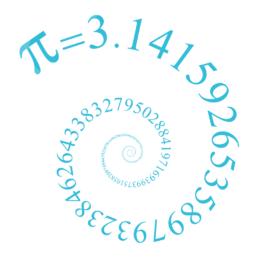Bournemouth Maths Tutor Barney M-T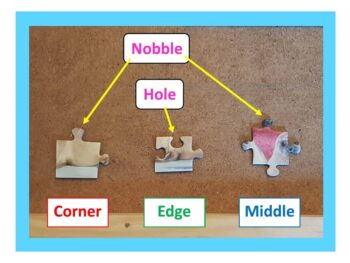HOW MUCH HARDER IS A 2000 PIECE JIGSAW THAN A 1000 PIECE JIGSAW?

First of all, it’s easy to see that a 2000 piece jigsaw is more than twice as hard as a 1000 piece jigsaw. To see why: imagine having two 1000 piece jigsaws that are of a similar style. Rather than complete them both separately (which would obviously be twice as hard as a 1000 piece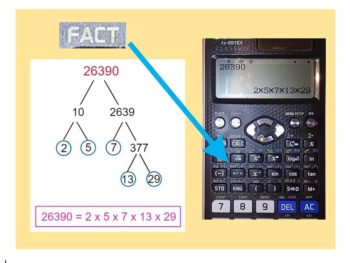PRODUCT OF PRIME FACTORS – A CALCULATOR SHORTCUT!

Expressing a positive integer (whole number) as the product of prime factors is a bit like dismantling a machine to see what it’s made of. Let’s try it with 26390. Drawing a Prime Factor Tree will take a while – but fortunately the calculator can do the hard work for us! Type in 26390, press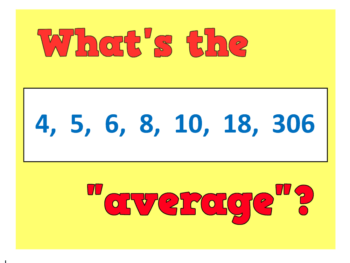WHAT IS THE “AVERAGE” IN MATHS?

An average is simply a way of picking out a typical value from a set of objects (usually numbers). There are infinitely many different ways to pick a “typical value”, but here are some of my favourites: Midrange average: The simplest of the lot: just calculate the number that is half way between the smallest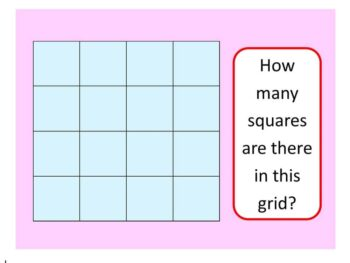HOW MANY SQUARES ARE THERE IN THIS GRID?

Before we see the quickest way to answer the question (scroll down if you are an expert!), let’s see some different possible approaches: GCSE APPROACH: There are 16 little squares.There is the one large square (the entire grid).But there are also some “2 by 2” squares, like the red one – I count 9 of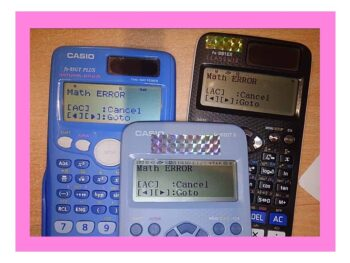What does “MATH ERROR” mean?

SHORT ANSWER: this means you have just asked the calculator to do a sum it doesn’t like. The three most common reasons for getting this error message are: 1) you have tried to divide by zero: forbidden in maths! If you type in $3\div 0$ you are asking the calculator “zero times by what equalsWHAT’S ZERO TIMES BY INFINITY?

SHORT ANSWER: 0 x ∞ = anything you like! Intrigued? Read on… LONG ANSWER: the question seems absurd: after all, zero multiplied by anything is zero, yet any multiple of infinity is always infinity. But 0 x ∞ cannot be both zero and infinity, can it? Infinity doesn’t behave in the same way as other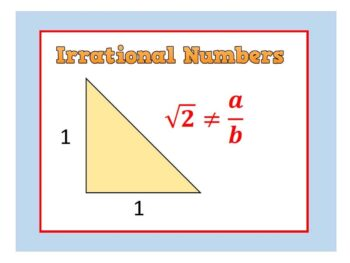Strange but True: IRRATIONAL NUMBERS

The first numbers we discover in childhood are the Natural Numbers: 1, 2, 3, … Next come the Integers (whole numbers) – which also include Zero and the negatives: … -3, -2, -1, 0, 1, 2, 3, … Next up: it’s fractions such as: 0.5, 2.90909… , $-\Large{5}\frac{7}{8}$ Numbers like these are said to beHere I am at the World’s Steepest Road in Harlech, North Wales. The road sign indicates an almighty 40% – but how is this measured? ON ROADS: The 40% means that however far you travel horizontally, you travel 40% of that vertically. So if you go 100m across, you go 40m up. SO A ROAD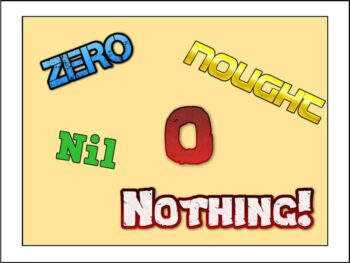(or: Much ado about Nothing) Zero doesn’t actually exist. Think about it: by definition it isn’t anything, it’s nothing!! Even so, here are 5 facts every mathematician should know about Zero: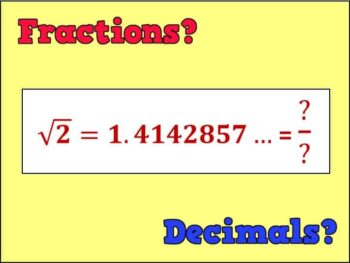WHICH ARE BETTER: FRACTIONS OR DECIMALS?

DECIMALS ARE WAAAY BETTER THAN FRACTIONS: most GCSE students prefer decimals because they allow you to compare the sizes of two numbers at a glance! For instance, which is bigger out of $\frac{2}{5}$ and $\frac{3}{7}$? Um…? But in decimal form we can easily see that $\frac{3}{7}=0.428571…>0.4=\frac{2}{5}$. FRACTIONS RULE SUPREME: fractions allow for easy multiplication, andQUOTE OF THE WEEK: “God Made the Integers. All Else is the Work of Man”

… or so thought Leopold Kronecker (1823-1891) in his famous quote. WHY KRONECKER WAS WRONG: the integers (whole numbers) include the Positive Integers or “counting numbers” 1, 2, 3, 4 and so on: easy for a child to understand. We could quite reasonably argue they are “made by God”. But the integers also include zero,FACTORIALS!

Factorials are so cool that the notation is: AN EXCLAMATION MARK!!!! The exclamation mark means something very specific in maths. It’s great to be impressed with numbers, but please do not put an exclamation mark after a number just to show that it’s really cool. WHAT IS IT? n Factorial (written $n!$) means the product

• 1
• 2